10 Images
 In:=Out=You can apply functions to images just like you apply functions to numbers or lists or anything else. The function ColorNegate that we saw in connection with colors also works on images, giving a negative image.
 In:=Out=In:=Out=In:=Out=You can make a table of the results of different amounts of blurring:
 In:=Out=ImageCollage puts images together:
 In:=Out=Theres lots of analysis one can do on images. For example, DominantColors finds a list of the most important colors in an image.
 In:=Out=Binarize makes an image black and white:
 In:=Out=Not surprisingly, the dominant colors in the result are black and white:
 In:=Out=Pick out edges in the original image:
 In:=Out=In:=Out=Its often convenient to do image processing interactively, creating interfaces using Manipulate. For example, Binarize lets you specify a threshold for what will be turned black as opposed to white. And often the best way to find the right threshold is just to interactively experiment with it.
Make an interface to adjust the threshold for binarizing an image:
 In:=Out=CurrentImage[ ] capture the current image from your computer, etc. ColorNegate[] negate the colors in an image Binarize[] convert an image to black and white Blur[, 5] blur an image EdgeDetect[] detect the edges in an image DominantColors[] get a list of dominant colors in an image ImageCollage[{,,}] put together images in a collage ImageAdd[,] add color values of two images
10.1Color negate the result of edge detecting an image. (Use CurrentImage[ ] or any other image.) »
Sample expected output:
 Out[]=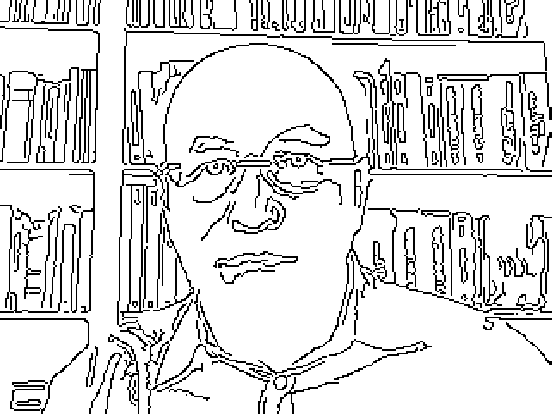Answer & check your solution
10.2Use Manipulate to make an interface for blurring an image from 0 to 20. »
Sample expected output:
 Out[]=Answer & check your solution
10.3Make a table of the results from edge detecting an image with blurring from 1 to 10. »
Sample expected output:
 Out[]=Answer & check your solution
10.4Make an image collage of an image together with the results of blurring, edge detecting and binarizing it. »
Sample expected output:
 Out[]=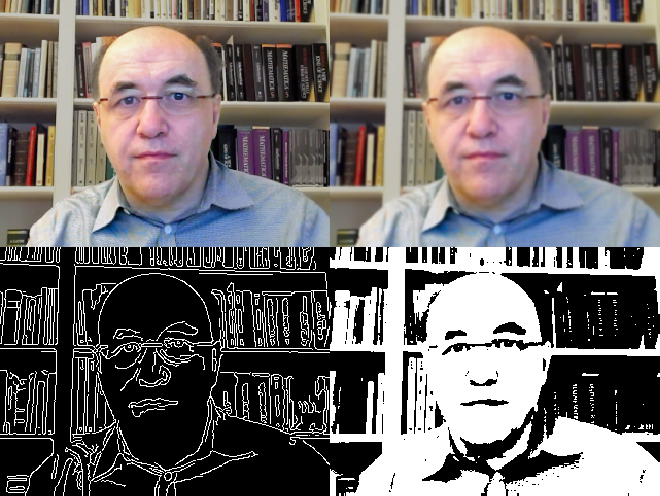Answer & check your solution
10.5Add an image to a binarized version of it. »
Sample expected output:
 Out[]=Answer & check your solution
10.6Create a Manipulate to display edges of an image as it gets blurred from 0 to 20. »
Sample expected output:
 Out[]=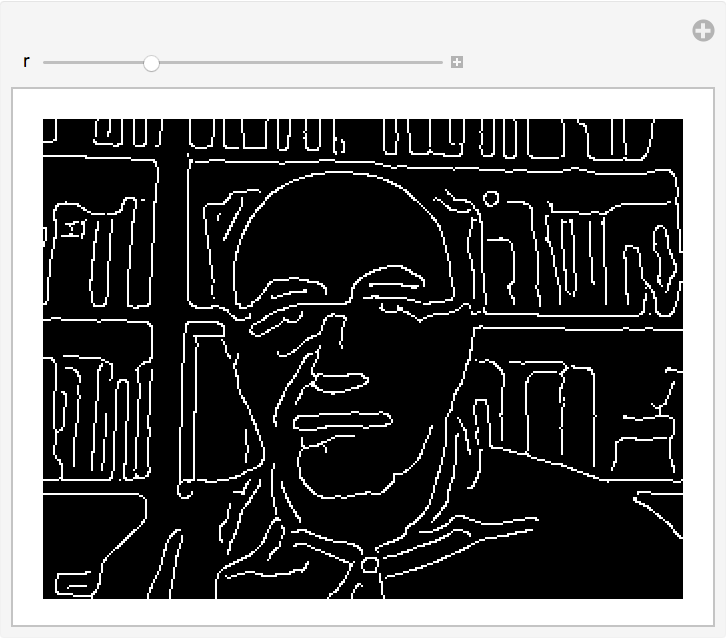Answer & check your solution
10.7Image operations work on Graphics and Graphics3D. Edge detect a picture of a sphere. »
Expected output:
 Out[]=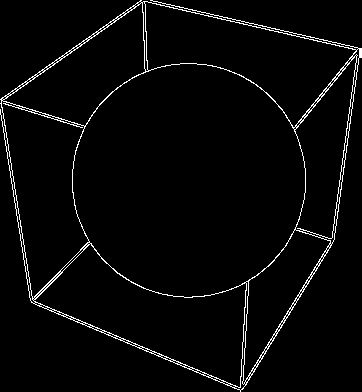Answer & check your solution
10.8Make a Manipulate to make an interface for blurring a purple pentagon from 0 to 20. »
Expected output:
 Out[]=Answer & check your solution
10.9Create a collage of 9 images of disks, each with a random color. »
Sample expected output:
 Out[]=Answer & check your solution
10.10Use ImageCollage to make a combined image of spheres with hues from 0 to 1 in steps of 0.2. »
Expected output:
 Out[]=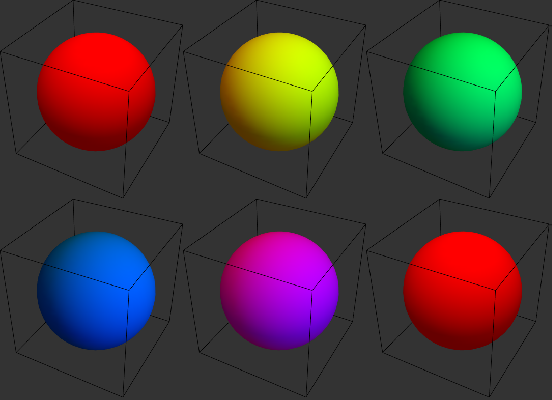Answer & check your solution
10.11Make a table of blurring a disk by an amount from 0 to 30 in steps of 5. »
Expected output:
 Out[]=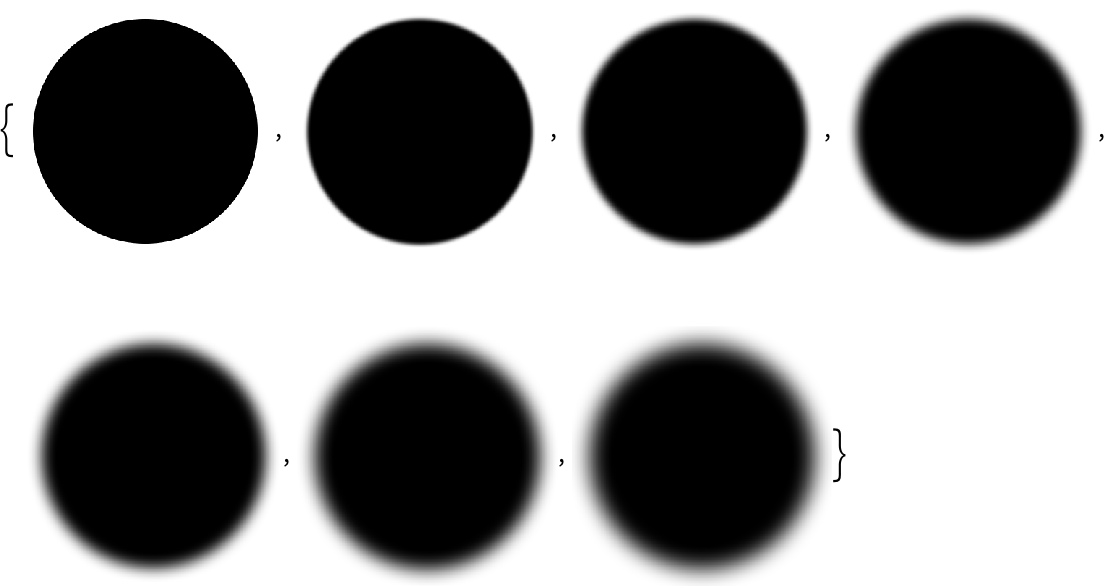Answer & check your solution
10.12Use ImageAdd to add an image to an image of a disk. »
Sample expected output:
 Out[]=Answer & check your solution
10.13Use ImageAdd to add an image to an image of a red octagon. »
Sample expected output:
 Out[]=Answer & check your solution
Sample expected output:
 Out[]=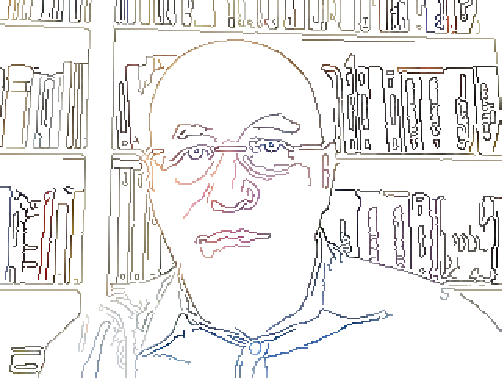Answer & check your solution
+10.1Edge detect a binarized image. »
Sample expected output:
 Out[]=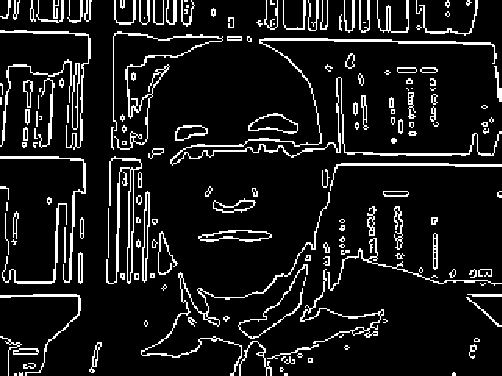Answer & check your solution
+10.2Color negate the result of edge detecting an image of a regular pentagon. »
Expected output:
 Out[]=Answer & check your solution
Sample expected output:
 Out[]=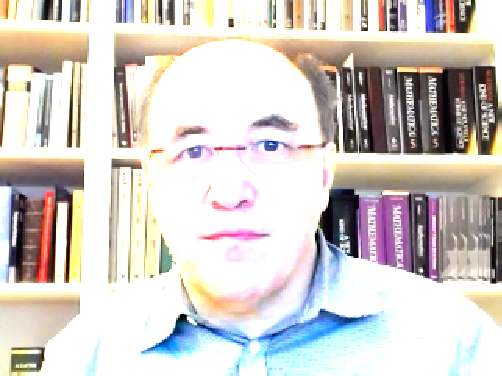Answer & check your solution
+10.4Use ImageAdd to add together the images of regular polygons with between 3 and 100 sides. »
Expected output:
 Out[]=Answer & check your solution
Instead of CurrentImage[], just get a test image using for example ExampleData[{"TestImage", "Mandrill"}].
What does the number in Blur mean?
Its the range of pixels that get blurred together.
How does Binarize decide whats black and whats white?
If you dont tell it a threshold, it will pick one based on analyzing the distribution of colors in the image.